# Math 3 C Systems of Differential Equations Autonomous

• Slides: 20Math 3 C Systems of Differential Equations Autonomous Examples Prepared by Vince Zaccone For Campus Learning Assistance Services at UCSBFind the nulllclines and equilibrium points for this system of differential equations. Prepared by Vince Zaccone For Campus Learning Assistance Services at UCSB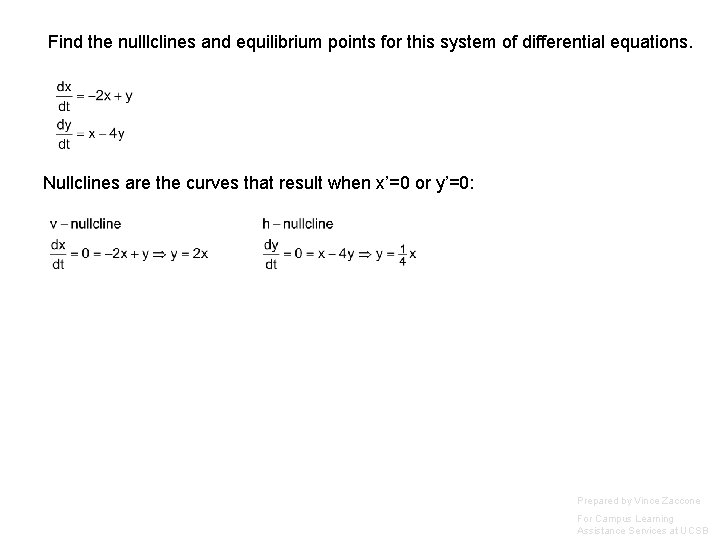Find the nulllclines and equilibrium points for this system of differential equations. Nullclines are the curves that result when x’=0 or y’=0: Prepared by Vince Zaccone For Campus Learning Assistance Services at UCSB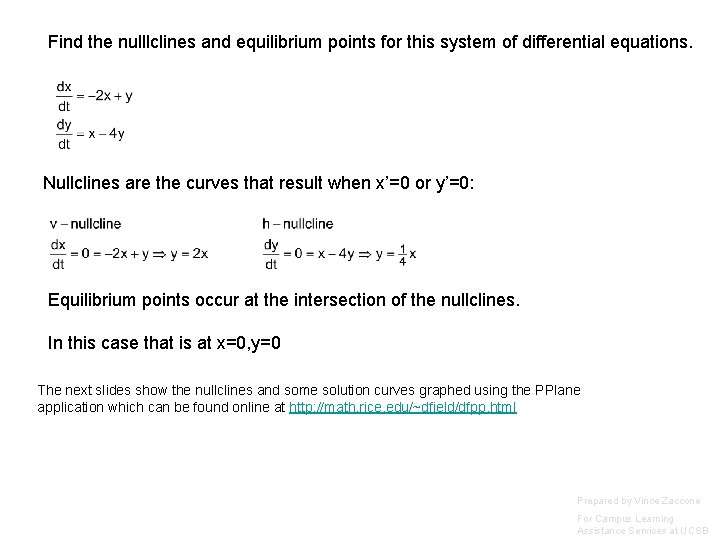Find the nulllclines and equilibrium points for this system of differential equations. Nullclines are the curves that result when x’=0 or y’=0: Equilibrium points occur at the intersection of the nullclines. In this case that is at x=0, y=0 The next slides show the nullclines and some solution curves graphed using the PPlane application which can be found online at http: //math. rice. edu/~dfield/dfpp. html Prepared by Vince Zaccone For Campus Learning Assistance Services at UCSBNullclines for this system v-nullcline: y=2 x h-nullcline: y=x/4Here is the phase plane diagram. Notice the equilibrium point is where the nullclines intersect. Looks stable - all the arrows point toward it.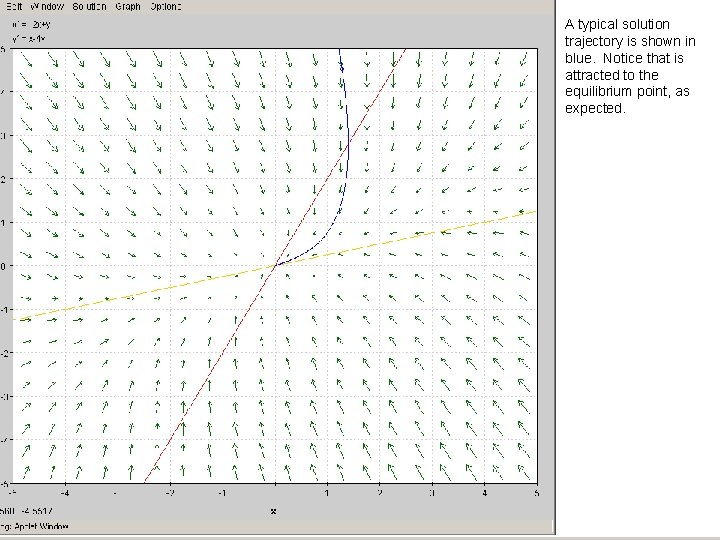A typical solution trajectory is shown in blue. Notice that is attracted to the equilibrium point, as expected.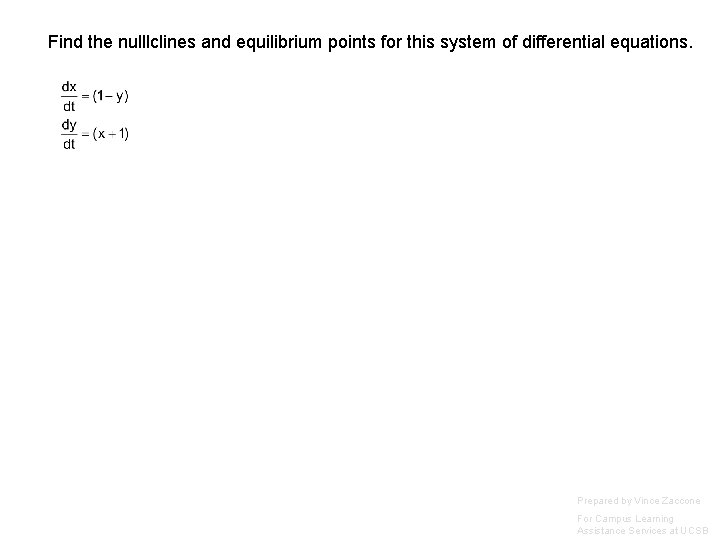Find the nulllclines and equilibrium points for this system of differential equations. Prepared by Vince Zaccone For Campus Learning Assistance Services at UCSB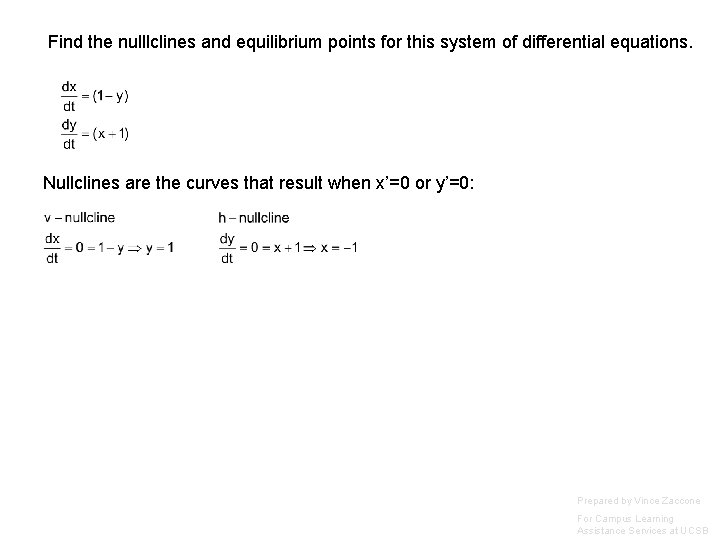Find the nulllclines and equilibrium points for this system of differential equations. Nullclines are the curves that result when x’=0 or y’=0: Prepared by Vince Zaccone For Campus Learning Assistance Services at UCSBFind the nulllclines and equilibrium points for this system of differential equations. Nullclines are the curves that result when x’=0 or y’=0: Equilibrium points occur at the intersection of the nullclines. In this case that is at x=-1, y=1 The next slides show the nullclines and some solution curves graphed using the PPlane application which can be found online at http: //math. rice. edu/~dfield/dfpp. html Prepared by Vince Zaccone For Campus Learning Assistance Services at UCSB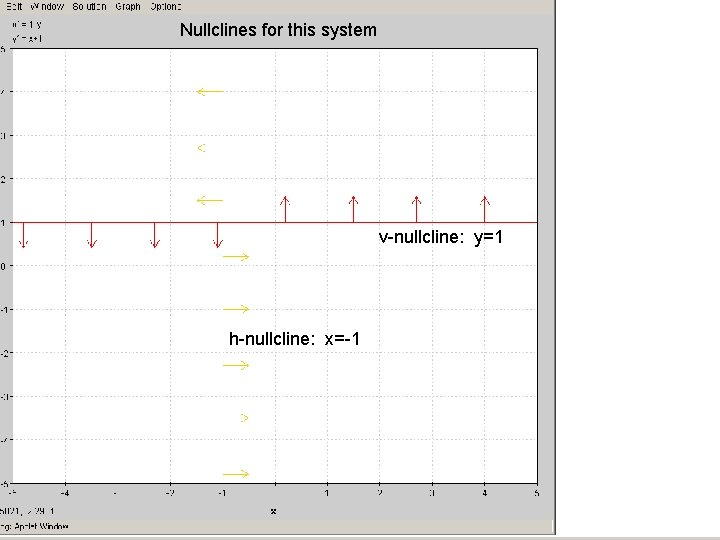Nullclines for this system v-nullcline: y=1 h-nullcline: x=-1Here is the phase plane diagram. Notice the equilibrium point is where the nullclines intersect.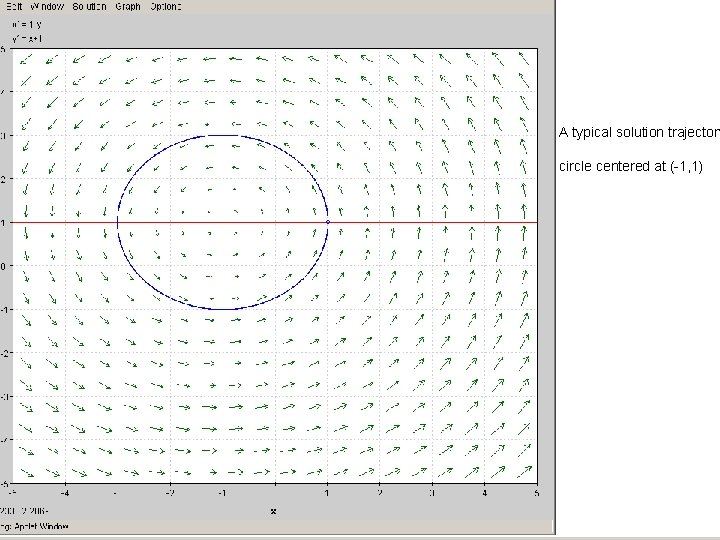A typical solution trajectory circle centered at (-1, 1)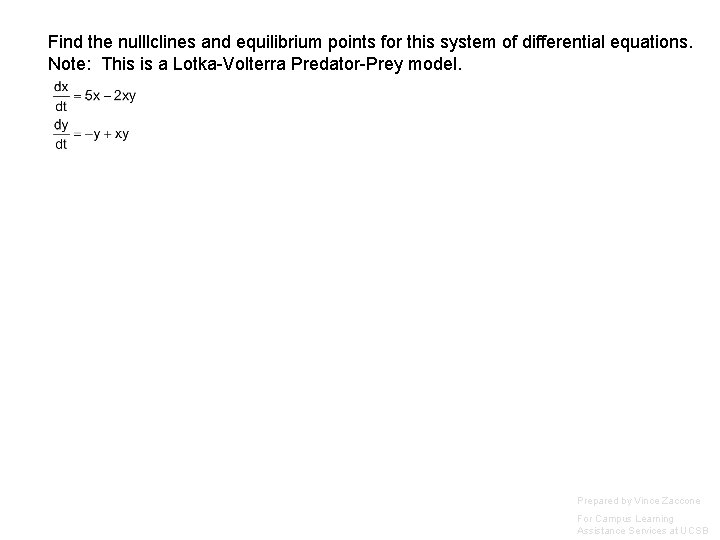Find the nulllclines and equilibrium points for this system of differential equations. Note: This is a Lotka-Volterra Predator-Prey model. Prepared by Vince Zaccone For Campus Learning Assistance Services at UCSBFind the nulllclines and equilibrium points for this system of differential equations. Note: This is a Lotka-Volterra Predator-Prey model. Prepared by Vince Zaccone For Campus Learning Assistance Services at UCSB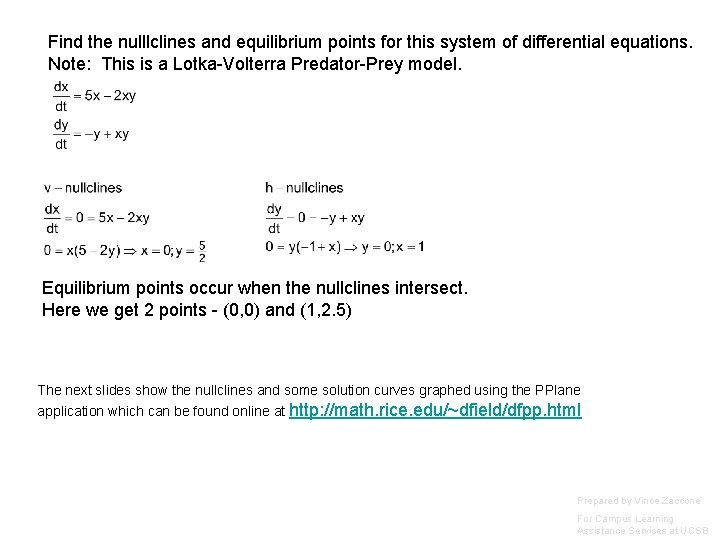Find the nulllclines and equilibrium points for this system of differential equations. Note: This is a Lotka-Volterra Predator-Prey model. Equilibrium points occur when the nullclines intersect. Here we get 2 points - (0, 0) and (1, 2. 5) The next slides show the nullclines and some solution curves graphed using the PPlane application which can be found online at http: //math. rice. edu/~dfield/dfpp. html Prepared by Vince Zaccone For Campus Learning Assistance Services at UCSB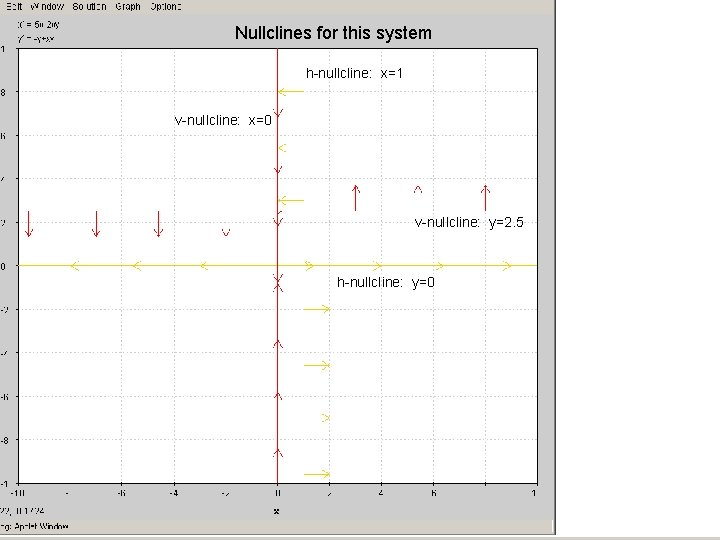Nullclines for this system h-nullcline: x=1 v-nullcline: x=0 v-nullcline: y=2. 5 h-nullcline: y=0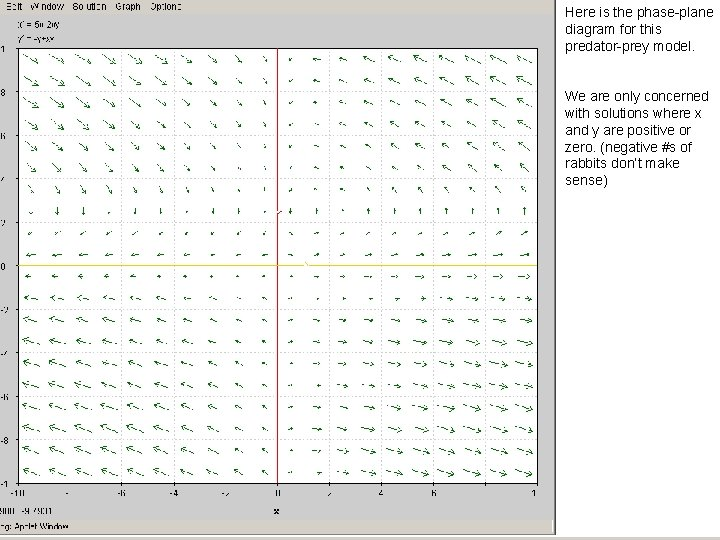Here is the phase-plane diagram for this predator-prey model. We are only concerned with solutions where x and y are positive or zero. (negative #s of rabbits don’t make sense)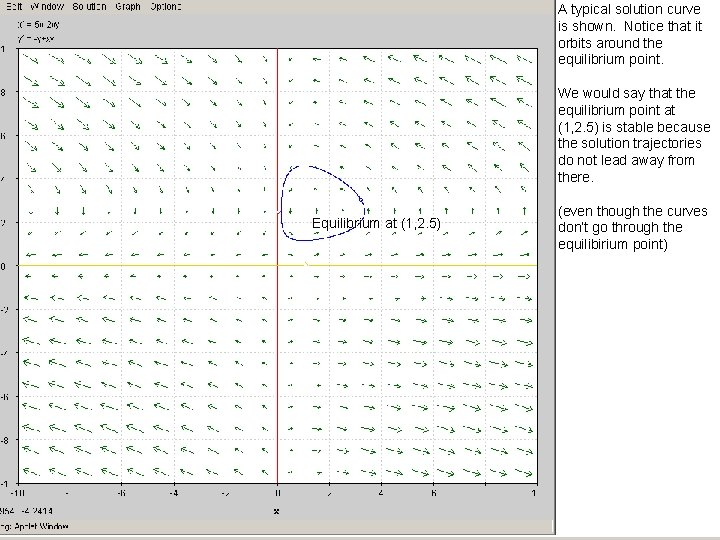A typical solution curve is shown. Notice that it orbits around the equilibrium point. We would say that the equilibrium point at (1, 2. 5) is stable because the solution trajectories do not lead away from there. Equilibrium at (1, 2. 5) (even though the curves don’t go through the equilibirium point)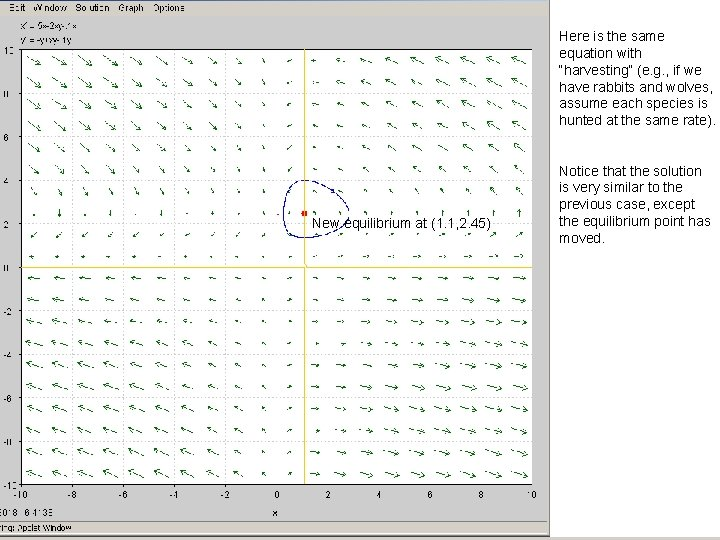Here is the same equation with “harvesting” (e. g. , if we have rabbits and wolves, assume each species is hunted at the same rate). New equilibrium at (1. 1, 2. 45) Notice that the solution is very similar to the previous case, except the equilibrium point has moved.